Phases (States) of Matter with Examples

Phases (States) of Matter with Examples

Matters can exist in four states; solid, liquid, gas and plasma. Some specific properties of these phases are given in the table below.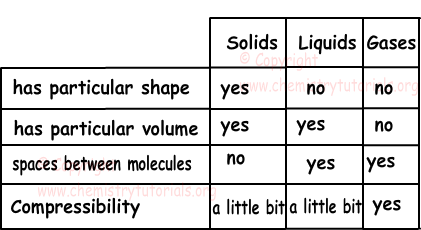In this unit we will examine solids, liquids and gases. Shape given below summarize the phase changes of matters from solid to liquid, liquid to gas.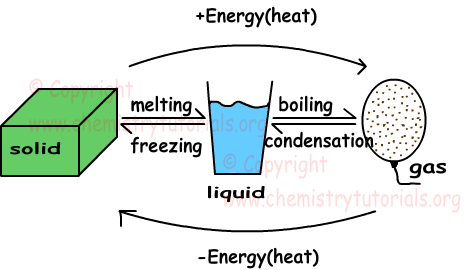Gaining heat in three phases of matter increase average kinetic energies of particles. For same matter kinetic energy of particles in gas phase is larger than the kinetic energies in solid and liquid states. Definitions of some concepts related to phase change are given below.

Melting: Solid matter changes its state to liquid.

Freezing: Opposite process of melting is called freezing. Liquid matter loses heat and changes its state to solid.

Boiling: Liquid matters gain heat and change their states to gas.

Condensation: Opposite process of boiling is called condensation. Gas molecules lose heat and change its phase to liquid.

During phase change, temperature of matters stay constant. Graphs of phase change are given below.

Temperature vs. time graph of heated pure solid substance is given below.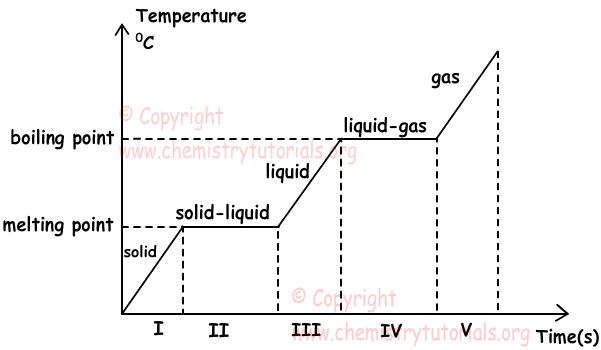In intervals I. III. and V. temperature of matter increases. Since matter is pure, kinetic energy of it also increases. We can find heat gained in these intervals with following formula;

Q=m.c.ΔT

where; m is mass, c is specific heat capacity and ΔT is change in the temperature (Tfinal-Tinitial)

In intervals II. and IV. temperature of matter stays constant because matter is changing phase. Since temperature of matter is constant, kinetic energy of it is also constant. On the contrary, during phase change, distances between molecules increase, thus potential energy of matter also increases. In these intervals, we have heterogeneous mixtures, for example in interval II. we have solid+liquid mixture and in interval IV. we have liquid+gas mixture. We find heat required in these intervals with following formulas;

Q=m.Lfusion or Q=m.Lvaporization

where, m is mass, Lfusion is latent heat of fusion and Lvaporization is latent heat of vaporization.

Losing heat of matter in gas phase has temperature vs. time graph as given below.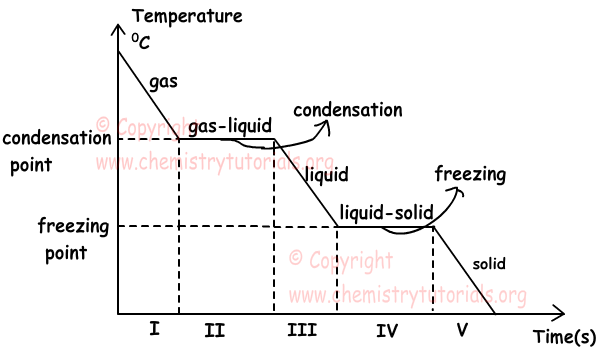As you can see in both of the graphs boiling point becomes condensation point and melting point becomes freezing point in cooling process. Their magnitudes are equal. We use same formulas given above to find heat released by matter during this process.

We solve some examples related to phase change.

Example: Find heat required to increase temperature of 100 g ice from 0C to 40 0C.(cwater=1cal/g0C, Lfusion=80cal/g)

Solution:

We melt ice first, then heat it to 40 0C.

Q1=m.Lfusion

Q1=100g.80.cal/g

Q1=8000 cal

Now we increase temperature from 0 to 400C

Q2=m.c.ΔT

Q2=100.1.(40-0)

Q2=4000cal

Qtotal=Q1+Q2=8000cal+4000cal=12000cal.

Example: If we mix two waters having mass 150 g and temperature 400C and 100g and 800C, find final temperature of mixtures.(cwater=1cal/g.0C)

Solution:

When two matters having different temperatures are in contact, there is a heat transfer between them. Heat flows from matter having higher temperature to matter having low temperature until they have equal temperatures. Heat gained is always equal to heat lost.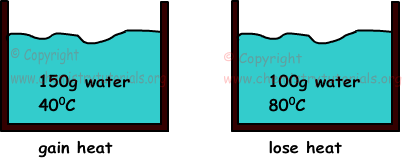Temperature of final mixture must be between temperatures of both waters

400C<Tfinal<800C

Qgained=Qlost

m1.c1.ΔT1=m2.c2.ΔT2

150.1.(T-40)=100.1.(80-T)

T=560C

Matters and Properties of Matters Exams and  Problem Solutions

Related